Course Content

0/4

0/3

0/1

0/2

0/1

0/4

0/3

0/5

0/3

0/5

0/5

0/1

0/6

0/2

0/4

0/5

2.3 The Relations of Roots and Coefficients of Quadratic Equations 一元二次方程式的根与系数的关系



If,are the roots of 3x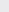– 2x – 3 = 0, find the values of,是 3x– 2x – 3 = 0 的根, 求值

[a][b][c](a)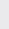(b)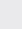(c)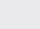The equation 3x+ 10x + p = 0, has rootsandwhere= 2, find the value of p.

3


The equation 2x– 2x + 3 = 0 has roots p and q and the equation x– x + 2m = 0 has roots and . Find the values of k and m.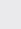,If the square of the difference of the roots of equation x– mx +15 = 0 is 4, find the value of m.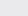Ifandare the roots of equation 2x– 3x+ 4 = 0, find the equation whose roots are是方程式2x– 3x+ 4 = 0 的根, 求作方程式其根是

[a],[b],(a) 8x– 18x + 13 = 0

(b) 4x+ 7x + 16 = 0



One root of the equation 2x– x + c = 0 is twice the other. Find the value of c.One root of the equation 3x– 3px + p= p + 6 is twice of the other. Find the values of p.

6, -3


Given thatandare the roots of the equation 2x= 3x – 4,

[a]

form an equation whose roots areand.

[b]

show that 4=– 12.

(a) 4x+ 23 = 0